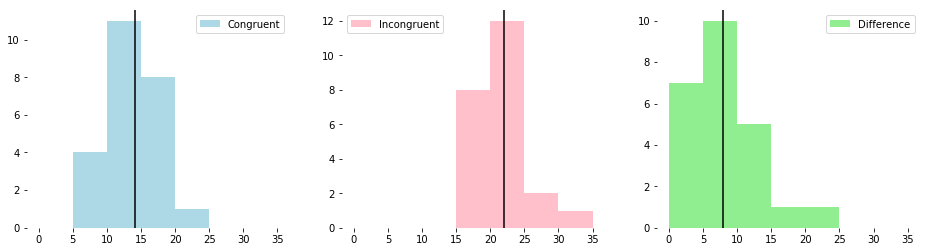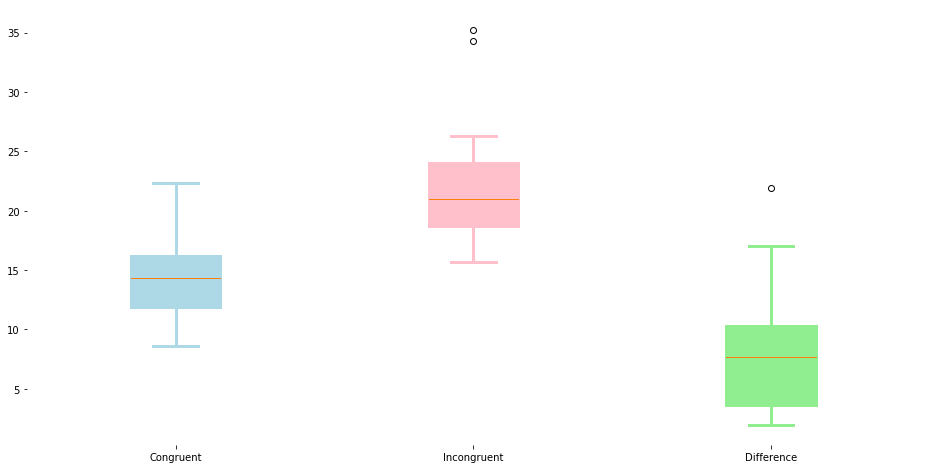### Analyzing the Stroop Effect¶

Perform the analysis in the space below. Remember to follow the instructions and review the project rubric before submitting. Once you've completed the analysis and write up, download this file as a PDF or HTML file and submit in the next section.

(1) What is the independent variable? What is the dependent variable?

Independent variable - which list is shown to the participant.

Dependent variable - the amount of time it takes the participant to name the ink colors for each list.

(2) What is an appropriate set of hypotheses for this task? What kind of statistical test do you expect to perform? Justify your choices.

Since we are trying to prove the presence of the stroop effect, the appropriate way to structure our set of hypotheses is to make our implicit assumption that the stroop effect does not exist.

That is, our alternative hypothesis (which we hope to prove) is that the mean difference between the time required to read the incongruent and congruent lists is greater than zero. Then, our null hypothesis (which we initially assume to be correct) is that the mean difference between the time required to read the incongruent and congruent lists is zero.

Recall that congruent means the ink color is the same as the written color, whereas incongruent means the ink color is different from the written color.

Written algebraically, the foregoing becomes the following...

$$H_0: \mu_{diff} = 0$$ $$H_A: \mu_{diff}> 0$$

where, $\mu_{diff}$ is defined as the average of the pairwise differences, $x_{diff}$:

$$x_{diff} = x_{incongruent}-x_{congruent}$$

Since the goal of this anlysis is to infer something about the population from our limited samples, the hypothesis is written in terms of the population mean, $\mu$.

The appropriate statistical test to perform is the "Dependent Samples T Test." This test is also known as the "Paired Samples T Test." This is the appropriate test because the data we have involves two measurements performed on the same person under different conditions, and contains no information about the population.

The fact that the samples are dependent is why also why the hypotheses are written in terms of the mean of differences, rather than the difference of means, although those approaches are very subtely different. Rarely would they produce different results.

(3) Report some descriptive statistics regarding this dataset. Include at least one measure of central tendency and at least one measure of variability. The name of the data file is 'stroopdata.csv'.

In :
# Perform the analysis here
import pandas as pd

print(df.shape)

(24, 2)

Out:
Congruent Incongruent
0 12.079 19.278
1 16.791 18.741
2 9.564 21.214
3 8.630 15.687
4 14.669 22.803
In :
df['difference'] = df['Incongruent'] - df['Congruent']

Out:
Congruent Incongruent difference
0 12.079 19.278 7.199
1 16.791 18.741 1.950
2 9.564 21.214 11.650
3 8.630 15.687 7.057
4 14.669 22.803 8.134
In :
cong_mean = df.Congruent.mean()
cong_std = df.Congruent.std()
incong_mean = df.Incongruent.mean()
incong_std = df.Incongruent.std()
diff_mean = df.difference.mean()
diff_std = df.difference.std()
diff_min = df.difference.min()

print( '  Congruent data -    mean :  ' + str(cong_mean) )
print( '  Congruent data - std dev :  ' + str(cong_std) )
print( '\r' )
print( 'Incongruent data -    mean :  ' + str(incong_mean) )
print( 'Incongruent data - std dev :  ' + str(incong_std) )
print( '\r' )
print( ' Difference data -    mean :  ' + str(diff_mean) )
print( ' Difference data - std dev :  ' + str(diff_std) )
print( ' Difference data -     min :  ' + str(diff_min) )

  Congruent data -    mean :  14.051125
Congruent data - std dev :  3.55935795765

Incongruent data -    mean :  22.0159166667
Incongruent data - std dev :  4.79705712247

Difference data -    mean :  7.96479166667
Difference data - std dev :  4.86482691036
Difference data -     min :  1.95


See results above.

The fact that there are no negative values in the difference data, as well as the mean and standard deviation of the difference data, imply that we should expect a result that will reject the null, and thereby confirm the presence of the Stroop effect.

(4) Provide one or two visualizations that show the distribution of the sample data. Write one or two sentences noting what you observe about the plot or plots.

In :
# Build the visualizations here
import matplotlib.pyplot as plt
%matplotlib inline

fig, axs = plt.subplots(1, 3, figsize=(16,4))
bins = [0,5,10,15,20,25,30,35]
colors = ['lightblue', 'pink', 'lightgreen']
means = [cong_mean, incong_mean, diff_mean]

axs.hist(df.Congruent, label='Congruent',
color=colors, bins=bins);

axs.hist(df.Incongruent, label='Incongruent',
color=colors, bins=bins);

axs.hist(df.difference, label='Difference',
color=colors, bins=bins);

for i, ax in enumerate(axs):
ax.axvline(means[i], color='black');
ax.legend();
ax.spines['top'].set_visible(False)
ax.spines['bottom'].set_visible(False)
ax.spines['right'].set_visible(False)
ax.spines['left'].set_visible(False)The three histograms above are plotted on a common x-axis. The values of the incongruent data appear to be generally higher than the congruent data.

These histograms appear to provide further indication that the Stroop effect was present, and that we should expect to reject the null hypothesis.

In :
fig, ax = plt.subplots(1, 1, figsize=(16,8))

bp = ax.boxplot([df.Congruent, df.Incongruent, df.difference],
labels = ['Congruent', 'Incongruent', 'Difference'],
patch_artist = True);

ax.spines['top'].set_visible(False)
ax.spines['bottom'].set_visible(False)
ax.spines['right'].set_visible(False)
ax.spines['left'].set_visible(False)

for i, box in enumerate(bp['boxes']):
box.set(color = colors[i], linewidth = 3)

for i, whisker in enumerate(bp['whiskers']):
whisker.set(color = colors[i//2], linewidth = 3)

for i, cap in enumerate(bp['caps']):
cap.set(color = colors[i//2], linewidth = 3)

for i, flier in enumerate(bp['caps']):
flier.set(color = colors[i//2], linewidth = 3)The box plots, above, provide another way to visualize the information in the histograms.

(5) Now, perform the statistical test and report the results. What is the confidence level and your critical statistic value? Do you reject the null hypothesis or fail to reject it? Come to a conclusion in terms of the experiment task. Did the results match up with your expectations?

In :
# Perform the statistical test here
from scipy import stats

diff_standard_error = diff_std / ( df.shape**0.5 )
t_stat = diff_mean / diff_standard_error

print('    T-stat for test data = {}'.format(t_stat))
print('P-value for given t-stat = {}'.format( \
1-stats.t.cdf(t_stat, df.shape-1)))

    T-stat for test data = 8.020706944109957
P-value for given t-stat = 2.0515002918664038e-08


For this analysis, we adopt the standard $\alpha$, or "Significance Level," of 0.05. This is the probability of rejecting the null hypothesis when it is actually true. Another value, called the "Confidence Level," is 1 minus $\alpha$, expressed as a percentage. For a significance level of 0.05, the confidence level is 95%.

The T-stat for our test data is 8.02, which, for 23 degrees of freedom, corresponds to a P-value less than 0.0000001. Recall that the P-value is the strength of evidence in support of the null hypothesis. Since the P-value is less than the significance level, we reject the null hypothesis.

Having taken this particular test myself before, I am not surprised by the results. Even with significant concentration it is difficult to quickly say the ink color a word is written in when that word itself spells out a color.